## slader A uniform cylindrical grinding wheel of mass 50.0 kg and diameter 1.0 m is turned on by an electric motor. The friction in the bearin

Question

slader A uniform cylindrical grinding wheel of mass 50.0 kg and diameter 1.0 m is turned on by an electric motor. The friction in the bearings is negligible. What torque must be applied to the wheel to bring it from rest to 120 rev/min in 20 revolutions?

in progress 0
2 months 2021-08-05T10:24:29+00:00 1 Answers 2 views 0

The value of torque on the rod is = 3.94 N – m

Explanation:

mass = 50 kg

Moment of inertia ( I ) =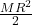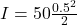I = 6.25 kg-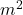Angular velocity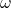= 120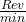= 12.6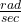Δ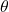= No. of rev × 2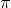Δ= 20 × 2Δ= 126 rad

From work energy theorem

Work done is equal to change in kinetic energy.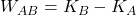——– (1)

Where A & B represents the initial & final states.

Since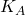= 0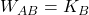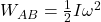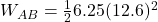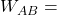496 J

We know thatT ΔWherework done

T = torque applied on the rod

Δ= Angular displacement of the rod.

496 = T × 126

T = 3.94 N – m

Therefore the value of torque on the rod is = 3.94 N – m